﻿ [SDOI2015]寻宝游戏 题解 – Hzao's Blog

# [SDOI2015]寻宝游戏 题解

### 题意分析

(在机房只能用Windows 7的画图工具，只能说还算能看吧)

### 解决方案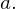假如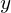在原有路径上，即加入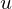之前，就被使节点连通的路径覆盖。也就是说，满足情况2或情况3。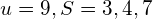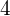能到达的 最近的 子树中有节点在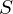中的 点。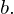假如不在原有路径上，那么以的子树外一定没有节点在中。（否则满足情况3，原有路径一定经过可知，无论选择哪个点，都有一段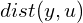，而这段距离是已固定的。（当前只有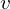不确定）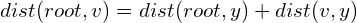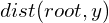是恒定的。

### 代码实现

1.节点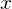的子树中有多少节点属于2.离节点最近的 子树中有节点属于的 点；

3.树上任意两点间的距离；

4.已经被路径覆盖的点中，距离根最近的点。的子树中的点就是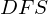序在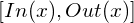中间的点。

（又巧了，倍增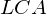不正好维护了每个节点的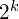倍祖先么？）

#include<cmath>
#include<queue>
#include<cstdio>
#include<cstring>

//#define LOC
#define nowy (L[i].to)
using std::queue;
using std::priority_queue;
template <typename T>
x=0;char c; T f=1;
do{c=getchar();if(c=='-')f=-1;}while(c>'9'||c<'0');
do{x=x*10+c-'0';c=getchar();}while(c>='0'&&c<='9');x*=f;
}

const int N=1e5 + 10;

int n,m,t;
int h[N],tot=1;
int f[N];
int d[N];
long long len[N];
int bg[N],ed[N];
int Inq[N];
int DFN;
int TotIn;
int Diff[N];//Diff是FT的一个拷贝 便于直接确认一个点是否在S中
int x,y,z;
int Num_SonIn,Cls_F;
long long ans=0;

struct line{
int to,nxt,w;
}L[2*N];

struct OneNode{
int num;
bool operator <(const OneNode b)const {
if(len[num]==len[b.num])return d[num]>d[b.num];
return len[num]>len[b.num];
}
OneNode(int x):num(x){}
};//通过便于维护小根堆
struct FenwickTree{
int v[N];
inline void clear(){
memset(v,0,sizeof(v));
}
for(;x<=n;x+=x&-x){
v[x]+=y;
}
}
int res=0;
while(x>0){
res+=v[x];
x-=x&-x;
}
return res;
}
FenwickTree(){clear();}
}FT;
priority_queue<OneNode> OQ;

inline void add(int x,int y,int z){
L[++tot].to=y;L[tot].nxt=h[x];h[x]=tot;L[tot].w=z;
L[++tot].to=x;L[tot].nxt=h[y];h[y]=tot;L[tot].w=z;
}

void GetLCA_DFS(int x){
//DFS 同时求出祖先数组、距离根节点的距离、DFS序
bg[x]=++DFN;
for(int i=h[x];i;i=L[i].nxt){
if(d[nowy])continue;
d[nowy]=d[x]+1;
f[nowy]=x;
len[nowy]=len[x]+L[i].w;
for(int j=1;j<=t;++j){
f[nowy][j]=f[f[nowy][j-1]][j-1];
}
GetLCA_DFS(nowy);
}
ed[x]=DFN;
}
inline int LCA(int x,int y){
if(d[x]>d[y]){
x^=y;y^=x;x^=y;
}
//d[x]<=d[y]
for(register int i=t;i>=0;--i){
if(d[f[y][i]]>=d[x]){
y=f[y][i];
}
}
if(x==y)return x;
for(register int i=t;i>=0;--i){
if(f[y][i]!=f[x][i]){
y=f[y][i];
x=f[x][i];
}
}
return f[x];
}
inline long long LEN(int x,int y){
return 1ll*len[x]+len[y]-2*len[LCA(x,y)];
}
inline int GetSonIn(int x){//including x
//判断x的子树中有多少节点属于S
return Num;
}
inline bool TwoSon(int x,int v){
//是否满足情况2
int SonV;
for(register int i=h[x];i;i=L[i].nxt){
if(d[nowy]<=d[x])continue;
SonV=GetSonIn(nowy);

if(SonV){
if(SonV==v)return false;
return true;
}
}
return false;
}
inline bool check(int x){
//是否满足情况2或3 这两种情况可以一同处理
if(!x)return true;
int Num_SonIn;
return (Num_SonIn=GetSonIn(x))&&(Num_SonIn<TotIn||TwoSon(x,Num_SonIn));
}
inline int GetCls_F(int x){
//倍增求离自己最近的 子树里有节点属于S 的节点
if(GetSonIn(x))return x;
int Num;
for(register int i=t;i>=0;--i){
if(!(Num=GetSonIn(f[x][i]))){
x=f[x][i];
}
}
return f[x];
}
inline void Change(long long t){
//通过t变量实现增加删除在同一个函数处理 减少码量
if(TotIn!=0){
if(!check(x)){
Cls_F=GetCls_F(x);
if(check(Cls_F)){
if(t&&!Inq[Cls_F]){OQ.push(OneNode(Cls_F));Inq[Cls_F]=1;}
ans+=LEN(x,Cls_F)*(-1+2*t);
}
else {
while(!OQ.empty()){
z=OQ.top().num;
if(check(z)||Diff[z])break;
OQ.pop();
Inq[z]=0;
}
if(t&&!Inq[Cls_F]){OQ.push(OneNode(Cls_F));Inq[Cls_F]=1;}
ans+=LEN(x,z)*(-1+2*t);
}
}
}
}
int main(){
t=log(n)/log(2)+1;
for(register int i=1;i<n;++i){
}
d=1;
GetLCA_DFS(1);
for(register int i=1;i<=m;++i){
if(!Diff[x]){
Change(1);
Diff[x]+=1;
if(!Inq[x]){Inq[x]=1;OQ.push(OneNode(x));}
++TotIn;
}
else{
--TotIn;
Diff[x]-=1;

Change(0);
}
printf("%lld\n",ans*2);
}
return 0;
}

Uae牛逼！